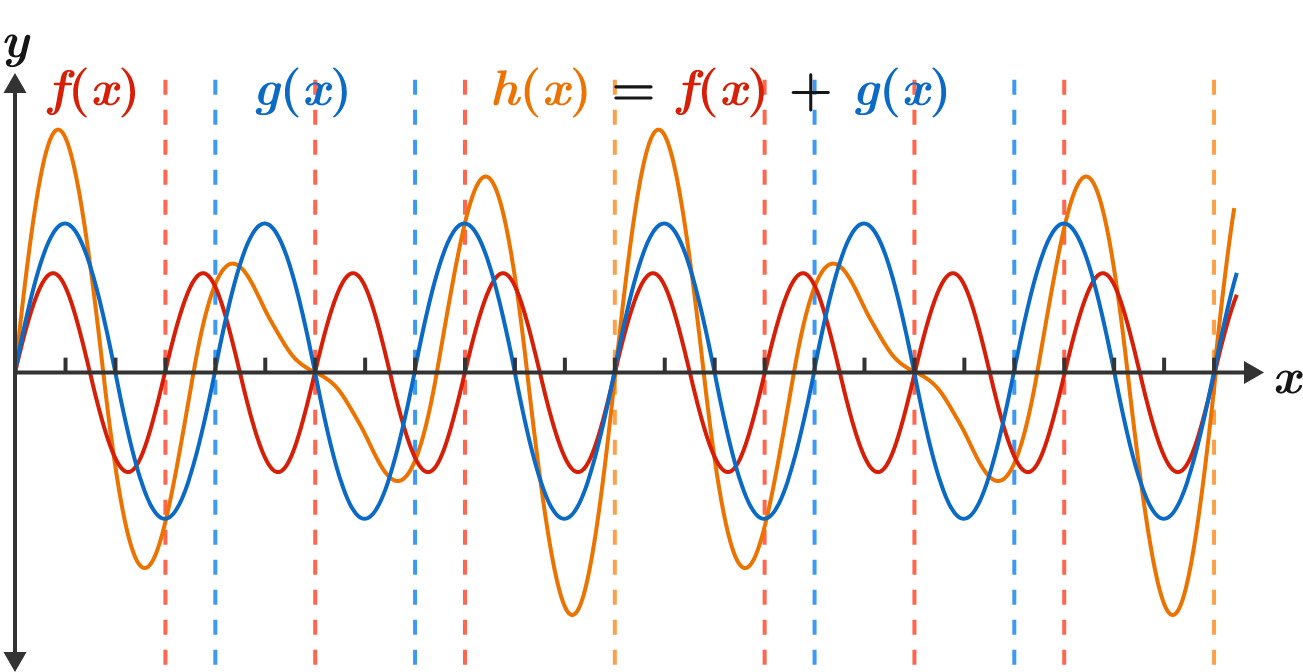# Period, period

Algebra Level 2

$\color{#D61F06}f(x),$ $\color{#3D99F6}g(x),$ and the function ${\color{#EC7300}h(x)}={\color{#D61F06}f(x)}+{\color{#3D99F6}g(x)}$ have fundamental periods of $3,$ $4,$ and $\text{lcm}(3,4)=12,$ respectively.

If $p(x)$ and $q(x)$ have fundamental periods $2$ and $\pi,$ respectively, what is the fundamental period of $r(x)=p(x)+q(x)?$×